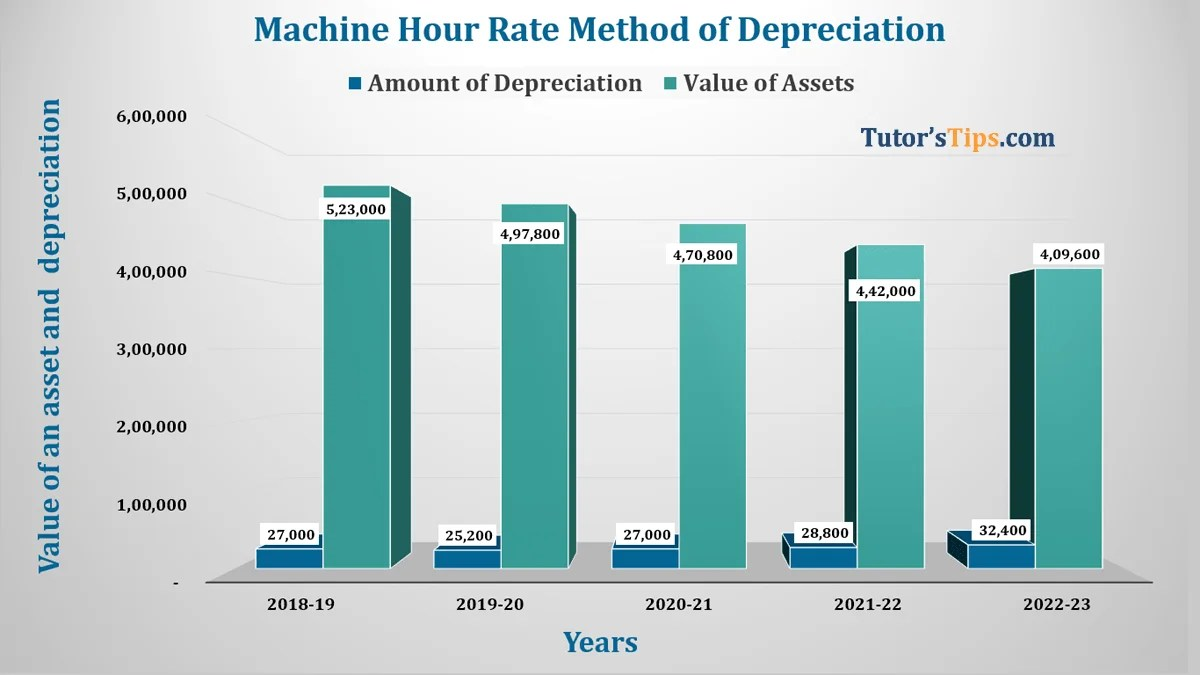# Machine Hour Rate Method of Depreciation | ExampleMachine hour rate Method of depreciation Feature image

Machine Hour Rate Method of Depreciation is applicable to those assets of which we can record their life in hours like Plant and Machine. So we have to keep the record of the total working hour of a machine consumed in a single financial year and the total amount of working hours consumed within the financial year is depreciation for that financial year. We will calculate depreciation by firstly calculate Machine Hour Rate then we multiply it by total working hours of the machine within a single financial year.

### This method of depreciation is applied in the following cases: –

• Where we can calculate the accurate life of an asset in hours.
• Where the life of an asset is certain.

### Example of Revaluation Method of Depreciation:

A Machine was purchased on 1st April 2018 at a cost of Rs 5,00,000/- and the cost of installation Rs 50,000/- and it can be disposed of for Rs 50,000/-. The expected total working life of the machine will be 1,00,000 hours. The following table shows the total working hours of the machine during the particular year in the next 5 years.

1. 31/03/2019 – 2100
2. 31/03/2020 – 1950
3. 31/03/2021 – 2200
4. 31/03/2022 – 2500
5. 31/03/2023 – 2350

Calculate the amount of depreciation.

Solution : –

Calculation of amount of depreciation under Machine Hour Rate Method of Depreciation:-

Formula:-

So now, we will calculate the amount of depreciation with above shown formula:

Total Cost of an asset                   =  5,00,000+50,000

=  5,50,000/-

Scrap value of an asset                =  50,000/-

Total life of an asset(in hours)    =  1,00,000 hours

So,

Depreciation(per hour)               =  (5,00,000+50,000)-50,000 / 1,00,000

=   Rs. 5 per hour

So now, the following table shows the calculation of an amount of depreciation year by year:-

 Year Total working hour(per year) Rate of Depreciation (Per Hour) Amount of Depreciation for Year 2018-19 5400 5 27,000 2019-20 5040 5 25,200 2020-21 5400 5 27,000 2021-22 5760 5 28,800 2022-23 6480 5 32,400